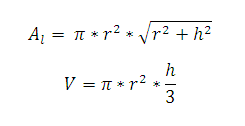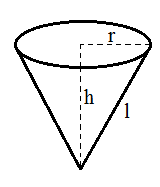# Right Circular Cone Calculator

This CalcTown calculator calculates the volume, lateral surface area of right circular cone.

#### Result

m3
m2Click here to view image

Where,

π = 3.14 (constant)

r = radius of the cone

h = height of the cone

V = volume of the cone

Al = lateral surface area of the cone